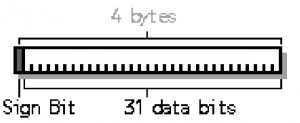Saturday, September 23, 2023 20:58

## Int variable type

A variable is a name that the compiler assigns to one or more memory location(s). When you declare a variable in a program, you have to specify its type and name. The type of a variable specifies the kind of values that the variable can store and the set of operations that the program can execute on variables. For the int variable type, the C# compiler usually allocates 32 bits (4 bytes). The int variable type can store values from -2,147,483,648 to 2,147,483,647. The following image illustrates how an int variable type is represented in C#:Int variable types are integer numbers. They do not have a fractional part, like real numbers (represented in floating point). If you assign to an int variable a value outside the  -2,147,483,648 to 2,147,483,647 range, you will get what programming calls overflowand the assigned value will be wrong.

The concepts explained in this lesson are also shown visually as part of the following video:

EXERCISES
1. Declare two variables of type int. Assign to them values 5 and 10 respectively. Exchange (swap) their values and print them.

Solution

Use third temporary variable for exchanging the variables:

To swap integer variables other solutions exist which do not use a third variable. For example, if we have two integer variables a and b:

You might also use the XOR swap algorithm for exchanging integer values: http://en.wikipedia.org/wiki/XOR_swap_algorithm.

2. The gravitational field of the Moon is approximately 17% of that on the Earth. Write a program that calculates the weight of a man on the moon by a given weight on the Earth.

Solution

Guidelines: Read the number from the console, multiply it by 0.17 and print it.

3. Write a program that takes as input a four-digit number in format abcd (e.g. 2011) and performs the following actions:
– Calculates the sum of the digits (in our example 2+0+1+1 = 4).
– Prints on the console the number in reversed order: dcba (in our example 1102).
– Puts the last digit in the first position: dabc (in our example 1201).
– Exchanges the second and the third digits: acbd (in our example 2101).

Solution

Guidelines: To get the individual digits of the number you can divide by 10 and take the remainder of the division by 10 four times.